# 知识蒸馏综述:网络结构搜索应用¶

【GiantPandaCV导语】知识蒸馏将教师网络中的知识迁移到学生网络，而NAS中天然的存在大量的网络，使用KD有助于提升超网整体性能。两者结合出现了许多工作，本文收集了部分代表性工作，并进行总结。

## 1. 引言¶

• 模型泛化能力更强

• 降低过拟合的风险

• 一定程度上降低了学生网络学习的难度。

• 对噪声更加鲁棒。

• 具有更多的信息量。

• 降低子网络收敛难度 ，在权重共享的NAS中，子网之间参数耦合，如果单纯用hard label训练，会导致互相冲突，导致子网络训练不足的情况。

• 如果引入额外的教师网络，可以带来更多的监督信息，加快网络的收敛速度

• 一些工作通过知识蒸馏引入评判子网的指标 ，比如与教师网络越接近的，认为其性能越好。

## 2. KD+NAS¶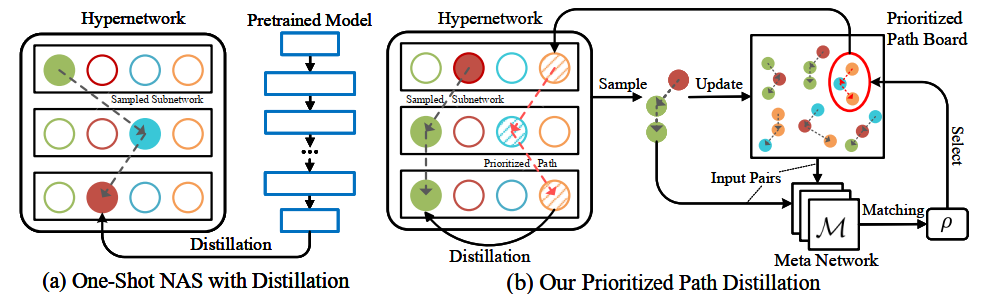• Prioritized Path Board : 这个模块中维护了一组优先路径，所谓优先路径就是性能表现较好的子网络，Cream将优先路径Board中的网络作为教师网络，引导知识的蒸馏。

• Meta Network: 这个模块是一个匹配模块，每当采样一个子网络，就会从优先路径Board中计算一个最匹配的网络作为教师网络，完成蒸馏的过程。

Cream中心思想是，子网络可以在整个训练过程中协作学习并相互教导，目的是提高单个模型的收敛性。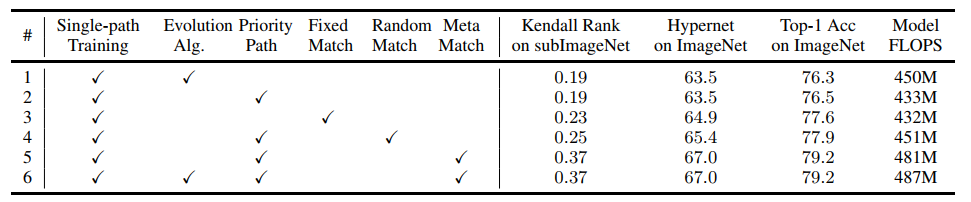### 2.2 DNA : Block-wisely Supervised Neural Architecture Search with Knowledge Distillation¶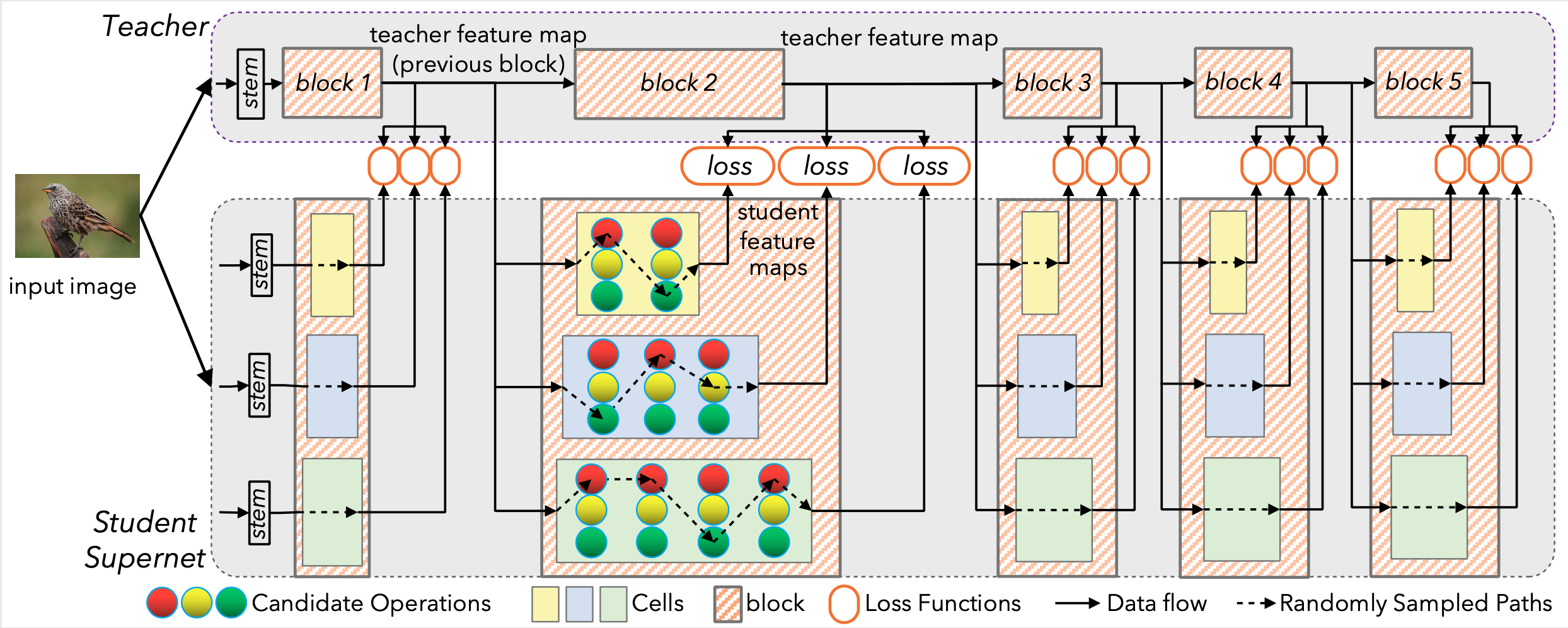DNA是两阶段的one-shot NAS方法，因此其引入蒸馏也是为了取代普通的acc指标，提出了使用子网络与教师网络接近程度作为衡量子网性能的指标。

### 2.3 AlphaNet :Improved Training of Supernet with Alpha-Divergence¶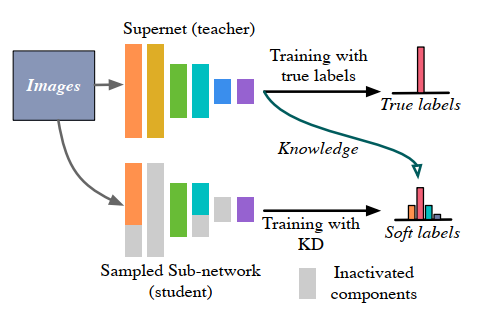\mathrm{KL}(p \| q)=\mathbb{E}_{p}[\log (p / q)]
• 避零性：zero avoiding 当p>0的时候，为了保证KL为正，q必须大于0。如果p=0的时候，q大于0也不会被惩罚。会导致下图所示的过估计问题over estimate。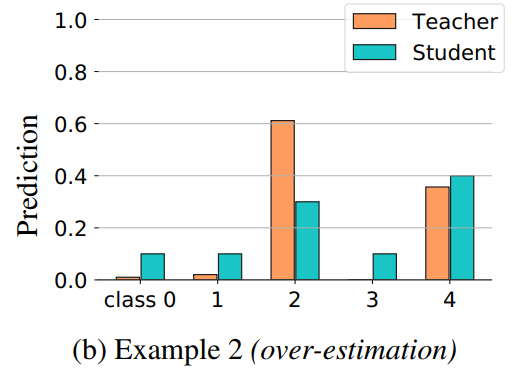• 零强制性：zero forcing 当p=0的时候，会强制q=0，因为如果q>0会导致KL divergence趋于无穷。会导致下图所示的低估问题under-estimate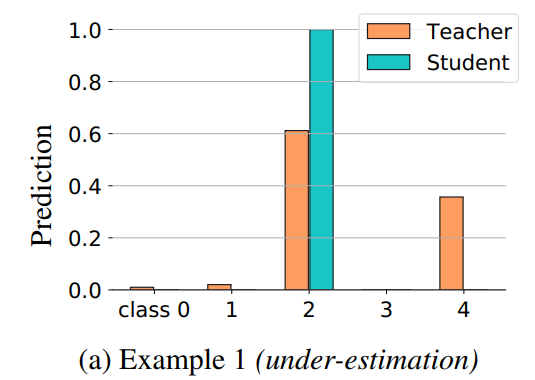AlphaNet提出了一个新的散度衡量损失函数，防止出现过估计或者低估的问题。如下所示，引入了$\alpha$

D_{\alpha}(p \| q)=\frac{1}{\alpha(\alpha-1)} \sum_{i=1}^{m} q_{i}\left[\left(\frac{p_{i}}{q_{i}}\right)^{\alpha}-1\right]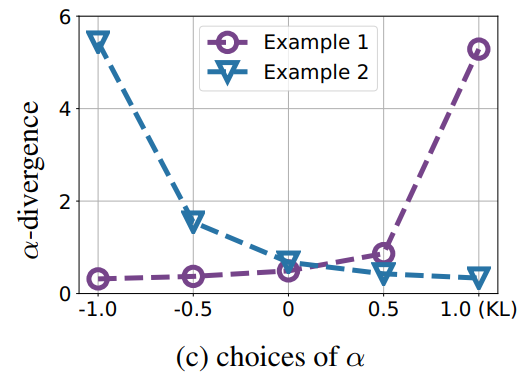D_{\alpha_{+}, \alpha_{-}}(p \| q)=\max \{\underbrace{D_{\alpha_{-}}(p \| q)}_{\begin{array}{c} \text { penalizing } \\ \text { over-estimation } \end{array}}, \underbrace{D_{\alpha_{+}}(p \| q)}_{\begin{array}{c} \text { penalizing } \\ \text { under-estimation } \end{array}}\}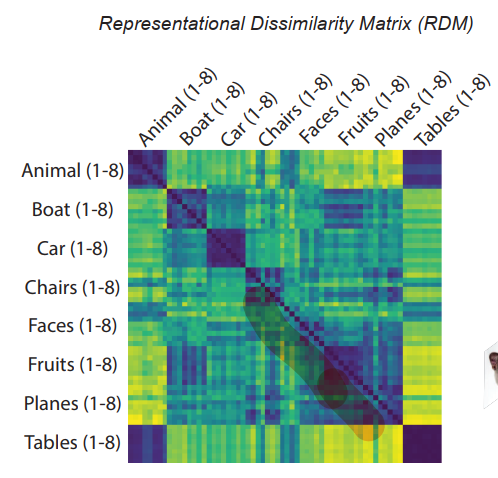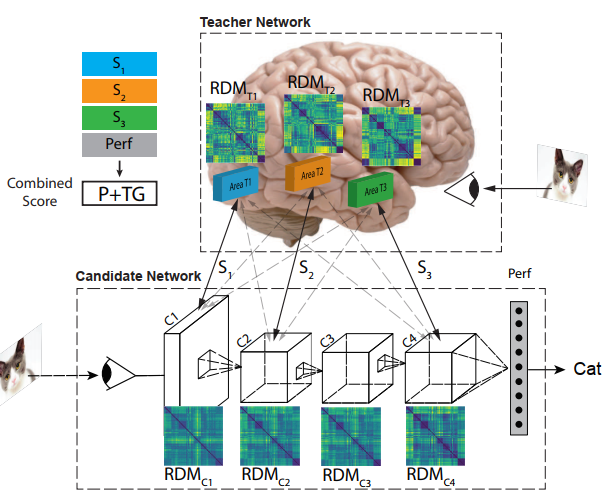• TG代表Teacher Guidance 计算的对象时所有RDM的均值。

• P代表Performance 也就是传统的准确率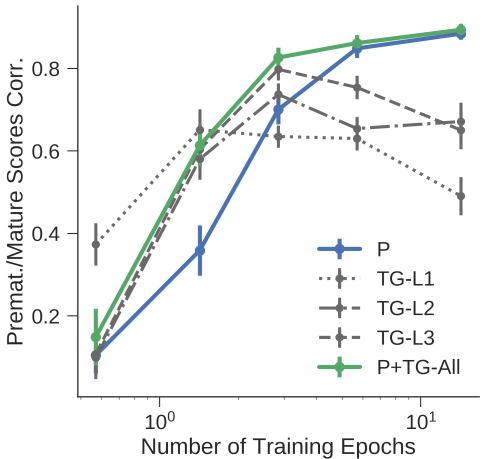### 2.5 Search for Better Students to Learn Distilled Knowledge¶

• 学生网络结构搜索 ：类似模型剪枝的方法，优化scale factor，然后剪枝的时候将该值较小的通道删除。

• 损失函数构建 ：下面与KD的区别是增加了对scale factor g的L1 Norm约束。

\min _{\mathbf{w}, \mathbf{g}} \frac{1}{N} \sum_{i=1}^{N} K L\left(f_{s}\left(\mathbf{x}_{\mathbf{i}}, \mathbf{w}, \mathbf{g}\right), f_{t}\left(\mathbf{x}_{\mathbf{i}}\right)\right)+\lambda_{1}\|\mathbf{w}\|_{2}+\lambda_{2} \sum_{j=1}^{M} \alpha_{j}\left\|g_{j}\right\|_{1}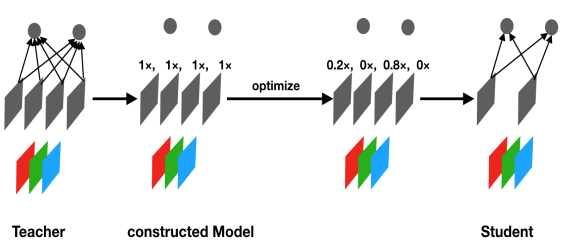### 2.6 Search to Distill: Pearls are Everywhere but not the Eyes¶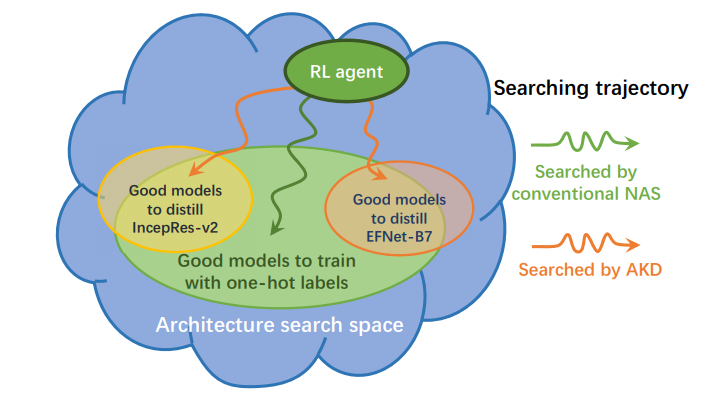Motivation: 先做了一组实验，发现不同的教师网络会倾向于不同的学生网络，因此在NAS中，使用不同的教师网络会导致模型倾向于选择不同的网络结构。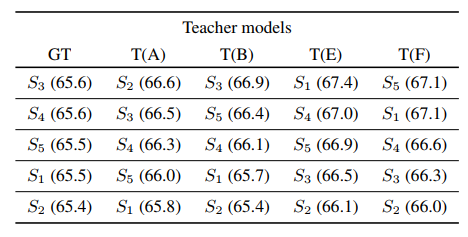AKD做法是选择使用强化学习的方法指导搜索过程， 使用的是ENAS那种通过RNN采样的方法。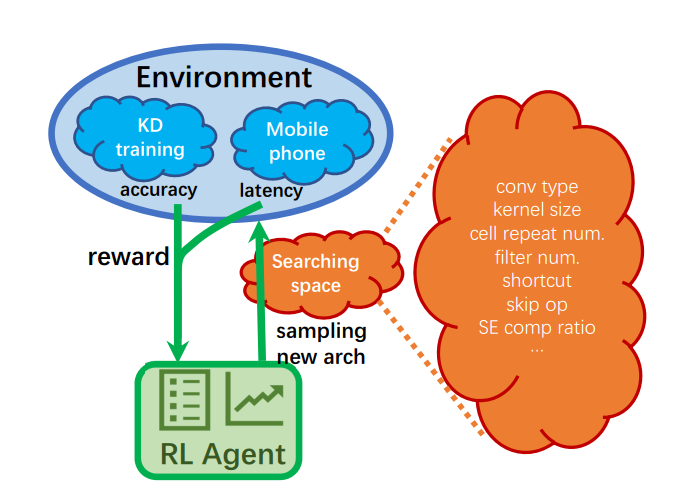### 2.7 Towards Oracle Knowledge Distillation with NAS¶

• 网络结构搜索 可以说占非常小的比重，因为搜索空间几乎属于微调，并不是从头搜索，而是大部分的结构都固定下来，只调整某些层的参数，用于控制模型容量。

• 知识蒸馏+模型集成 ：提出了动态选择待集成的模型，选择对应的教师网络进行蒸馏。

### 2.8 AdaNAS: Improving neural architecture search image classifiers via ensemble learning¶

• 使用集成的模型性能是否会提升

• 通过先前集成的模型指导模型蒸馏能否生效

• 能否得到一种自动化的集成模型的方式。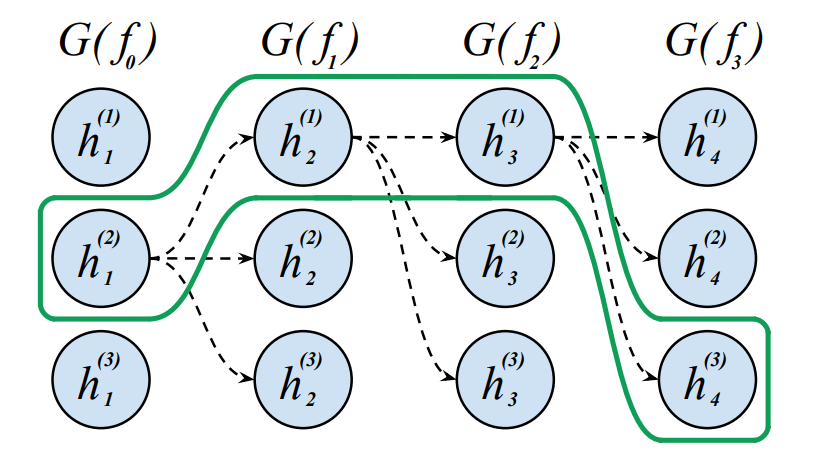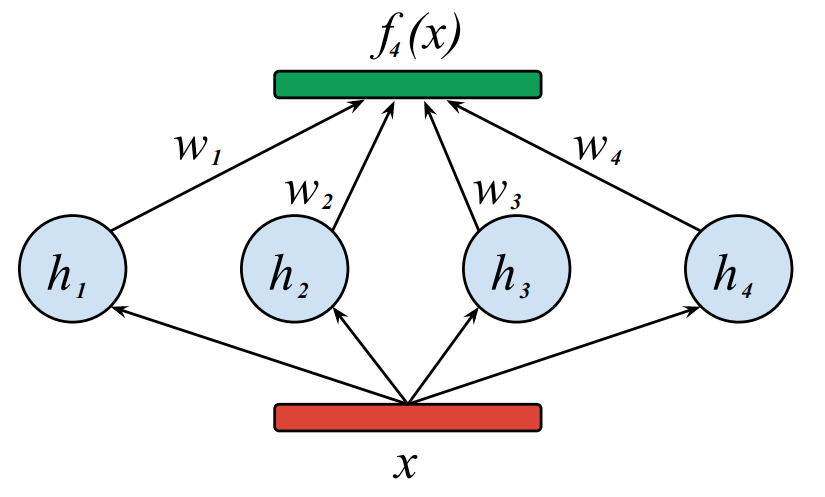f_{i}=\sum_{k=1}^{i} w_{k} \cdot h_{k}

### 2.9 Differentiable Feature Aggregation Search for¶

Knowledge Distillation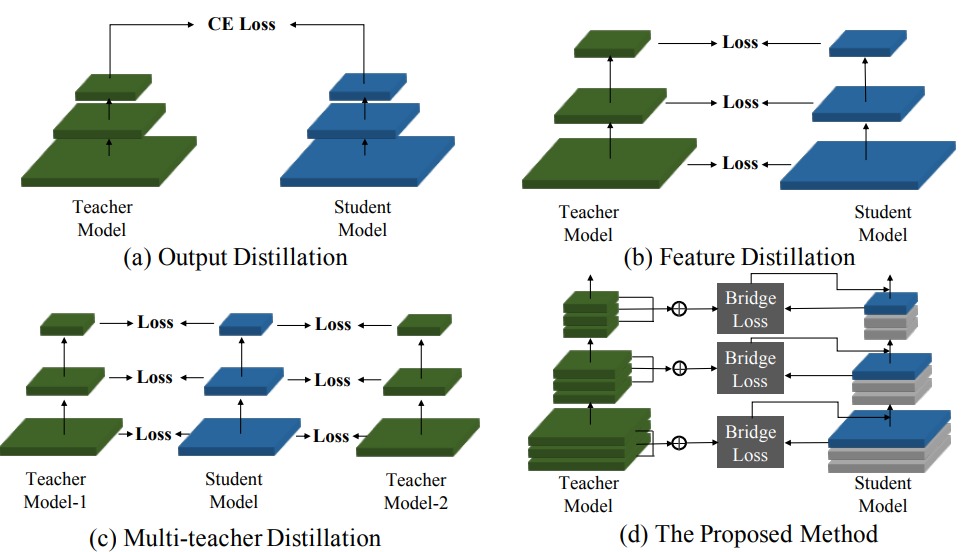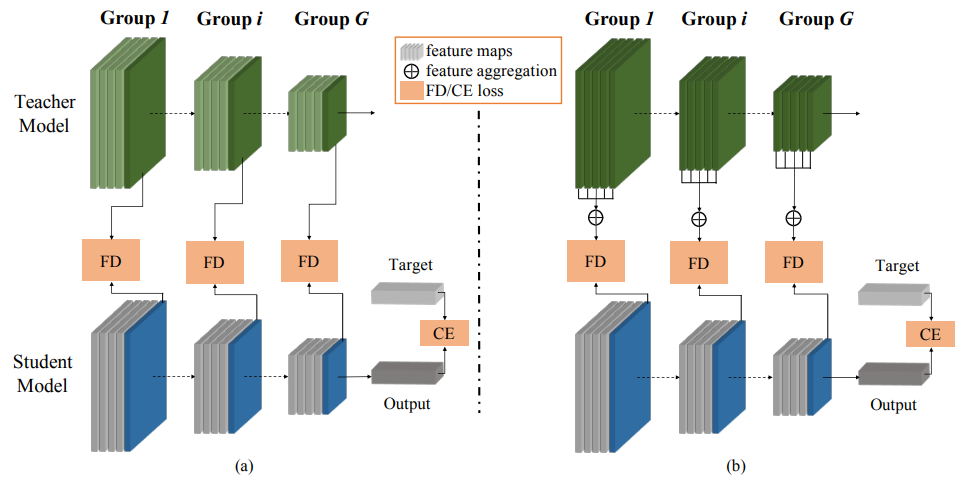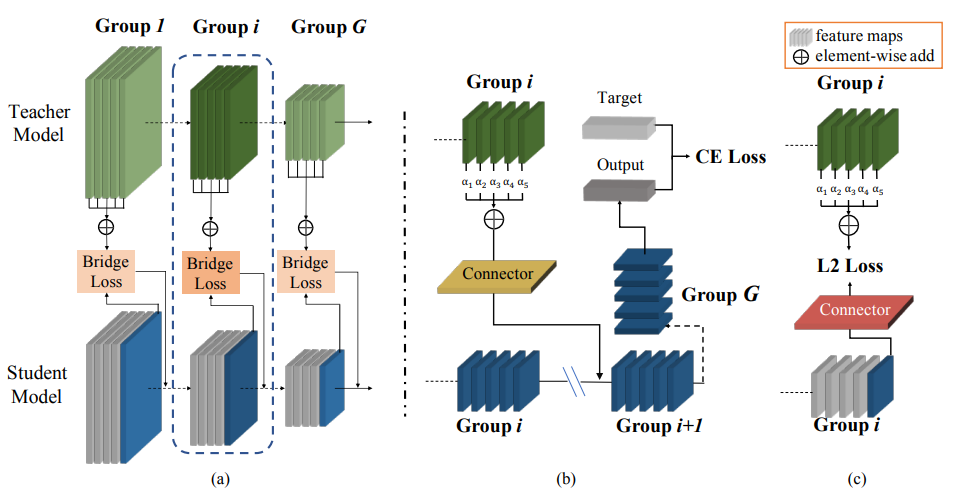（ps: connector让人想到VID这个工作）

• Circumventing Outliers of AutoAugment with Knowledge Distillation

• Improving Neural Architecture Search Image Classifiers via Ensemble Learning

• Multi-fidelity Neural Architecture Search with KD